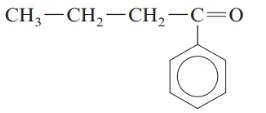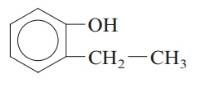Chapter 20, Problem 136AP### Introductory Chemistry: A Foundati...

9th Edition
Steven S. Zumdahl + 1 other
ISBN: 9781337399425

#### Solutions

Chapter
Section### Introductory Chemistry: A Foundati...

9th Edition
Steven S. Zumdahl + 1 other
ISBN: 9781337399425
Textbook Problem
1 views

# . On the basis of the functional groups listed in Table 20.5, identify the family of organic compounds to which each of the following belongs. a.b.c.d.Interpretation Introduction

(a)

Interpretation:

The family of organic compounds for the given compound should be determined.

Concept Introduction:

Functional group controls the physical and chemical properties of an organic compound.

Explanation

The given compound is:

Carboxylic acid is a class of organic compounds which has both carbonyl and hydroxyl group i...

Interpretation Introduction

(b)

Interpretation:

The family of organic compounds for the given compound should be determined.

Concept Introduction:

Functional group controls the physical and chemical properties of an organic compound.

Interpretation Introduction

(c)

Interpretation:

The family of organic compounds for the given compound should be determined.

Concept Introduction:

Functional group controls the physical and chemical properties of an organic compound.

Interpretation Introduction

(d)

Interpretation:

The family of organic compounds for the given compound should be determined.

Concept Introduction:

Functional group controls the physical and chemical properties of an organic compound.

### Still sussing out bartleby?

Check out a sample textbook solution.

See a sample solution

#### The Solution to Your Study Problems

Bartleby provides explanations to thousands of textbook problems written by our experts, many with advanced degrees!

Get Started